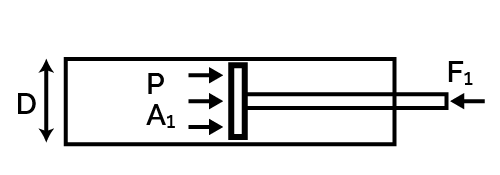# Pneumatic Cylinder Force Calculator

Created by Luis Hoyos
Last updated: Feb 18, 2023

Welcome to the pneumatic cylinder force calculator, a tool that, apart from calculating the cylinder force, you can use for other purposes, such as:

• Calculating the cylinder bore (diameter);
• Calculating the cylinder area;
• Calculating the cylinder pressure;

The following sections present the basic formulas this tool uses to calculate the cylinder force and how to calculate the cylinder tonnage. If you click on the advanced mode of the calculator, you can account for the percentage decrease that the friction force causes on the ideal force prediction of those formulas.

Be also sure to check the piston speed calculator to learn how to find piston speed using stroke and revolutions per minute!

## Single-acting pneumatic cylinder force calculation

In a single-acting pneumatic cylinder, the pressurized gas (typically air) enters through one port and returns to its initial position, usually with the aid of a spring.

The formula to calculate the pneumatic cylinder force is the same as for any other piston force calculation. The pressure inside the cylinder acts over the circular area of the piston. As we saw in our pressure calculator, this force equals the pressure times piston area:

F = P × A

where:

• F — Cylinder force, calculated in Newtons (N);
• P — Cylinder pressure, in Pascals (Pa);
• A — Piston area, in square meters ().

If we don't know the area of the piston, we can calculate it using the piston diameter (D):

A = πD²/4*

Then, the final formula for a single-acting cylinder is:

F = P × π/4

#### Formula to calculate the cylinder bore

We can solve for D and obtain a formula to calculate the cylinder bore as long as we know the cylinder pressure and the required force:

D = 4F/(πP)

#### The formula for cylinder pressure

We can also solve for P and obtain a formula for the cylinder pressure required as long as we know the cylinder bore and required force:

P = 4F/(πD²)

## Double-acting pneumatic cylinder force calculation

In a double-acting cylinder, instead of a spring, we use pressurized gas to achieve the return stroke and exert a force in an additional direction.

The force exerted in the outward stroke is the same as in a single-acting cylinder. In the return stroke, the force is slightly lower as the area in contact with the pressurized gas decreases because the rod occupies a portion of that area.

Then, the formulas for each stroke are:

• outFeffec = P × (π/4) × D² for the outward stroke, and
• returnFeffec = P × (π/4) × (D² - d²) for the return stroke.

## How do I calculate the cylinder tonnage?

The cylinder tonnage is simply the cylinder force expressed in tone-force units. To calculate the cylinder tonnage:

• If the force is in newtons, divide it by 9807; or
• If the force is in pounds-force, divide it by 2000.
Luis Hoyos
Cylinder type
Single-actingCylinder pressure (P)
psi
Piston diameter/bore (D)
in
Piston area (A₁)
in²
Cylinder force
Outward stroke force (F₁)
lbf
People also viewed…

### Brake Mean Effective Pressure (BMEP)

Determine the brake mean effective pressure of an IC engine with this BMEP calculator.